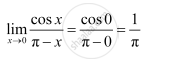CBSE (Arts) Class 11CBSE
Share

# Evaluate the Given Limit Lim_(X ->0) Cos X/(Pi - X) - CBSE (Arts) Class 11 - Mathematics

ConceptLimits of Trigonometric Functions

#### Question

Evaluate the given limit lim_(x ->0) cos x/(pi - x)

#### SolutionIs there an error in this question or solution?

#### APPEARS IN

NCERT Solution for Mathematics Textbook for Class 11 (2018 to Current)
Chapter 13: Limits and Derivatives
Q: 16 | Page no. 302

#### Video TutorialsVIEW ALL 

Solution Evaluate the Given Limit Lim_(X ->0) Cos X/(Pi - X) Concept: Limits of Trigonometric Functions.
S